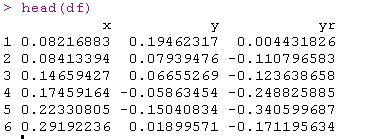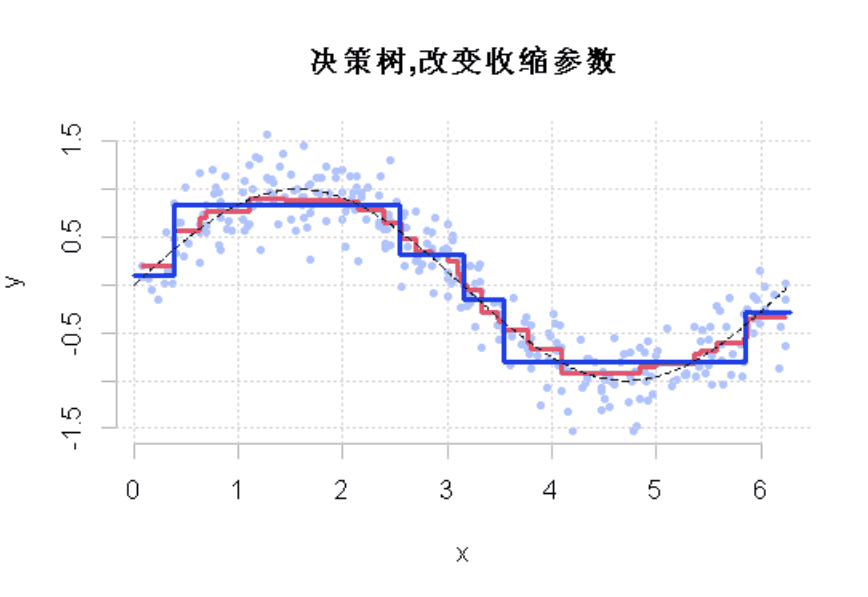Boosting算法是一种把若干个分类器整合为一个分类器的方法，也就是一种集成分类方法（Ensemble Method）

## 计量经济学的视角

Boosting集成学习原理与R语言提升回归树BRT预测短鳍鳗分布生态学实例

## 样条曲线``lsgen(x, y, degree = 1, numknot = 2)``

``````v=.05

fit=lm(y~bs(x,degree=1,knots=optknot))
yp=predict(fit,newdata=df)
yr= y - v*yp
YP=v*yp
for(t in 1:200){
fit=lm(yr~bs(x,degree=1,knots= optknot) )

plot(  x,  y,ylab="",xlab="")
lines(  x,y,type="l" )``````

``viz(100)``

# R语言基于树的方法：决策树，随机森林，Bagging，增强树

## 决策回归树

``````v=.1
rpart(y~x,data=df)
yp=predict(fit)
yr= y -  yp
YP=v*yp
for(t in 1:100){
predict(fit,newdata=df)``````

### 随时关注您喜欢的主题

`````` plot( x, y,ylab="",xlab="")
lines( x,y,type="s"
fit=rpart(y~x,data=df)``````

``````viz=function(v=0.05)
f\$yr=df\$y -v*yp
YP=v*yp
for(t in 1:100){
yp=predict(fit,newdata=df)
yr= yr - v*yp
lines(df\$x,y,type="s"``````# 显然，这个收缩参数有影响。它必须很小才能得到一个好的模型。这就是使用弱学习来获得好的预测的想法。

Ik是被错误分类的个体集合。

``````
for(i in 1:n_iter)rfit = rpart(y~., x, w, method="class")
g = -1 + 2*(predict(rfit,x)[,2]&gt;.5)
e = sum(w*(y*>0))
error[i] = mean(1*f*y<0)
plot(seq(1,n_iter),error

``````​

train_car = car[id_train,]
test_car= car[-id_train,]

​

# 在这里，我们面临一个机器学习中的经典问题：我们有一个完美的模型，误差为零。用多项式拟合：有10个观察值，9度的多项式，拟合很好。将我们的数据集一分为二，一个训练数据集，一个验证数据集。

``````for(i in 1:n_iter){

rfit = rpart(y\_train~., x\_train, w_train, method="class")
train\_error\[i\] = mean(1\*f\_train\*y_train&lt;0)
test\_error\[i\] = mean(1\*f\_test\*y_test&lt;0)}
plot(seq(1,n\_iter),test\_error)``````

## R函数：梯度提升(_GBM_)算法

``gbm(y~ .,n.trees = 200,shrinkage = .01,cv.folds = 5``

Kaizong Ye拓端研究室（TRL）的研究员。

​非常感谢您阅读本文，如需帮助请联系我们！QQ在线咨询

15121130882

0571-63341498

## 关注有关新文章的微信公众号

This will close in 0 seconds